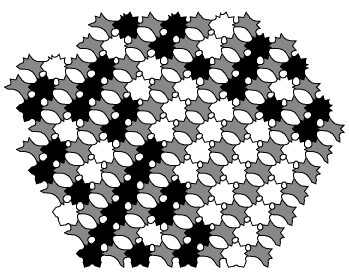Universal jigsaw puzzle

This is a Ghost Diagram tileset implementing Rule 110, a cellular automaton known to be capable of universal computation:

'a aC C', 'a bC D', 'b aD C', 'b bD D', 'cacAAA', 'dacBBB', 'cbcBBB', 'dbcBBB', 'cadAAA', 'dadBBB', 'cbdBBB', 'dbdAAA', colors=['#fff', '#000', '#888', '#888', '#888', '#888', '#fff', '#000', '#000', '#000', '#fff', '#000', '#000', '#fff']Were you to physically instantiate this tileset (very easy to do), the result would be a very simple universal computer in the form of a jigsaw puzzle. You could make it out of injection moulded plastic. You could sell it as a child's toy, a bucket full of computation.

One interesting thing about this tileset is that causality is defined by the tiles, rather than assumed (as in cellular automata). Ghost-diagrams has no idea which way it should be working, and has a great deal of difficulty solving the tileset -- it tends to try working backwards in time. (A smarter assembler could undoubtedly do better.)

The arrow of time in physics remains something of a mystery. Maybe this is the start of an explanation. It's easy to imagine tilesets that are only causal above some scale, or only mostly causal. There's a teasing similarity to Feynman diagrams, in which particles sometimes travel backwards in time. There are tilesets that look awfully like Feynman diagrams.

Here's code to generate other elementary cellular automata:

```rule = 110

result = [ "a aC C", "a bC D", "b aD C", "b bD D" ]
colors = [ "#fff", "#000" ] + ["#888"]*4

for i in xrange(8):
a = i&1
b = (i>>1)&1
c = (i>>2)&1
out = (rule>>i)&1
result.append("cd"[a] + "ab"[b] + "cd"[c] + "AB"[out]*3)
colors.append(["#fff","#000"][out])

print repr(result)[1:-1] + ", colors=" + repr(colors)```

 [æ]